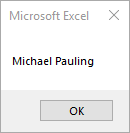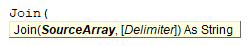## Join Description

Joins a number of substrings into a single string.

## Simple Join Examples

```Sub Join_Example()
Dim strFullName As String
Dim arrName(0 To 2) As String
arrName(0) = "Michael"
arrName(1) = "Pauling"
strFullName = Join(arrName)

MsgBox strFullName
End Sub```

This will display as following.## Join Syntax

In the VBA Editor, you can type  “Join(” to see the syntax for the Join Function:The Join function contains 2 arguments:

SourceArray: 1d array containing substrings to be joined.

Delimiter: [Optional] Delimiter. If omitted, the space character (” “) is used.

## Examples of Excel VBA Join Function

```Sub JoinArray_Example1()
Dim strFullPath As String
Dim arrPath(0 To 2) As String
arrPath(0) = "C:"
arrPath(1) = "Users"
arrPath(2) = "Public"
strFullPath = Join(arrPath, "\")
End Sub```

Result: C:\Users\Public

```Sub JoinArray_Example2()
MsgBox Join(Array("A", "B", "C"), ", ")
End Sub```

Result: “A, B, C”